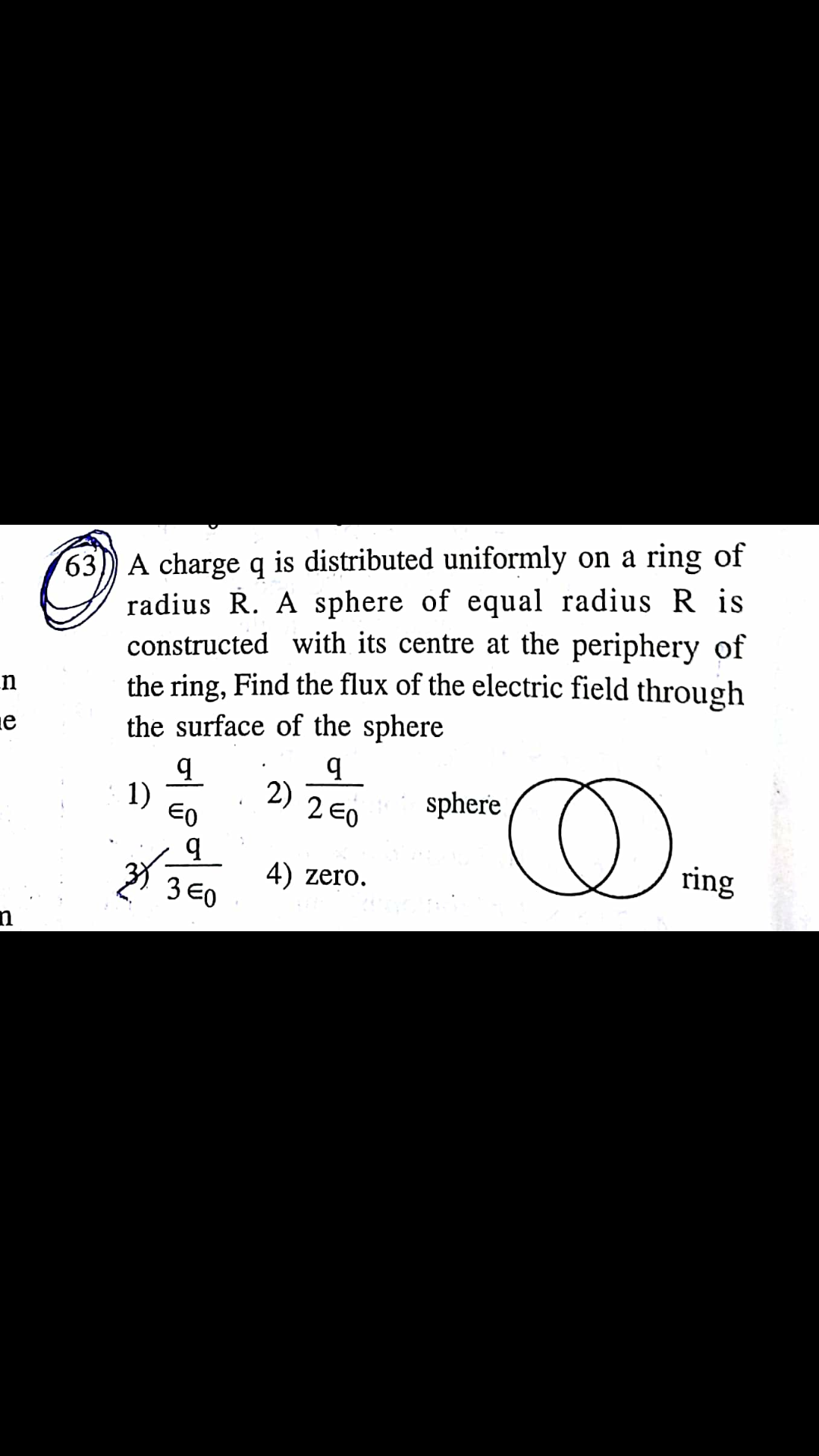# Good evening sir.This is Gauss law application.Explain it sir.4 years ago
Dear Raju

let point of intersection of ring & sphere are P,Q ...let center of ring is C & center of sphere is C1 ...

CC1 bisects PQ in two equal parts , if S is the point of intersection of CC1 & PQ then

in triangle right angled PCS

PC = R , CS = R/2 so angle PCS = @

using trigonometry , cos@ = CS/PS = 1/2

@ = pi/3

total angle substended by arc is 2pi/3...this is the arc of ring which lies inside sphere & substends

2pi/3 angle at the center of ring...

for 2pi radian charge  = q